# q-functions¶

## q-Pochhammer symbol¶

### qp()¶

mpmath.qp(a, q=None, n=None, **kwargs)

Evaluates the q-Pochhammer symbol (or q-rising factorial)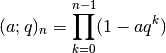where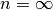is permitted if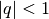. Called with two arguments, qp(a,q) computes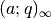; with a single argument, qp(q) computes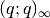. The special caseis also known as the Euler function, or (up to a factor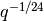) the Dedekind eta function.

Examples

If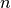is a positive integer, the function amounts to a finite product:

>>> from mpmath import *
>>> mp.dps = 25; mp.pretty = True
>>> qp(2,3,5)
-725305.0
>>> fprod(1-2*3**k for k in range(5))
-725305.0
>>> qp(2,3,0)
1.0


Complex arguments are allowed:

>>> qp(2-1j, 0.75j)
(0.4628842231660149089976379 + 4.481821753552703090628793j)


The regular Pochhammer symbol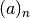is obtained in the following limit as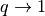:

>>> a, n = 4, 7
>>> limit(lambda q: qp(q**a,q,n) / (1-q)**n, 1)
604800.0
>>> rf(a,n)
604800.0


The Taylor series of the reciprocal Euler function gives the partition function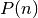, i.e. the number of ways of writingas a sum of positive integers:

>>> taylor(lambda q: 1/qp(q), 0, 10)
[1.0, 1.0, 2.0, 3.0, 5.0, 7.0, 11.0, 15.0, 22.0, 30.0, 42.0]


Special values include:

>>> qp(0)
1.0
>>> findroot(diffun(qp), -0.4)   # location of maximum
-0.4112484791779547734440257
>>> qp(_)
1.228348867038575112586878


The q-Pochhammer symbol is related to the Jacobi theta functions. For example, the following identity holds:

>>> q = mpf(0.5)    # arbitrary
>>> qp(q)
0.2887880950866024212788997
>>> root(3,-2)*root(q,-24)*jtheta(2,pi/6,root(q,6))
0.2887880950866024212788997


## q-gamma and factorial¶

### qgamma()¶

mpmath.qgamma(z, q, **kwargs)

Evaluates the q-gamma function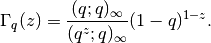Examples

Evaluation for real and complex arguments:

>>> from mpmath import *
>>> mp.dps = 25; mp.pretty = True
>>> qgamma(4,0.75)
4.046875
>>> qgamma(6,6)
121226245.0
>>> qgamma(3+4j, 0.5j)
(0.1663082382255199834630088 + 0.01952474576025952984418217j)


The q-gamma function satisfies a functional equation similar to that of the ordinary gamma function:

>>> q = mpf(0.25)
>>> z = mpf(2.5)
>>> qgamma(z+1,q)
1.428277424823760954685912
>>> (1-q**z)/(1-q)*qgamma(z,q)
1.428277424823760954685912


### qfac()¶

mpmath.qfac(z, q, **kwargs)

Evaluates the q-factorial,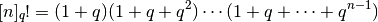or more generally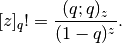Examples

>>> from mpmath import *
>>> mp.dps = 25; mp.pretty = True
>>> qfac(0,0)
1.0
>>> qfac(4,3)
2080.0
>>> qfac(5,6)
121226245.0
>>> qfac(1+1j, 2+1j)
(0.4370556551322672478613695 + 0.2609739839216039203708921j)


## Hypergeometric q-series¶

### qhyper()¶

mpmath.qhyper(a_s, b_s, q, z, **kwargs)

Evaluates the basic hypergeometric series or hypergeometric q-series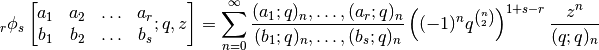wheredenotes the q-Pochhammer symbol (see qp()).

Examples

Evaluation works for real and complex arguments:

>>> from mpmath import *
>>> mp.dps = 25; mp.pretty = True
>>> qhyper([0.5], [2.25], 0.25, 4)
-0.1975849091263356009534385
>>> qhyper([0.5], [2.25], 0.25-0.25j, 4)
(2.806330244925716649839237 + 3.568997623337943121769938j)
>>> qhyper([1+j], [2,3+0.5j], 0.25, 3+4j)
(9.112885171773400017270226 - 1.272756997166375050700388j)


Comparing with a summation of the defining series, using nsum():

>>> b, q, z = 3, 0.25, 0.5
>>> qhyper([], [b], q, z)
0.6221136748254495583228324
>>> nsum(lambda n: z**n / qp(q,q,n)/qp(b,q,n) * q**(n*(n-1)), [0,inf])
0.6221136748254495583228324